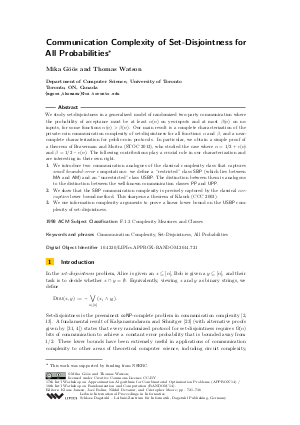Document# Communication Complexity of Set-Disjointness for All Probabilities

### Authors Mika Göös, Thomas Watson## File

LIPIcs.APPROX-RANDOM.2014.721.pdf
• Filesize: 0.71 MB
• 16 pages

## Cite As

Mika Göös and Thomas Watson. Communication Complexity of Set-Disjointness for All Probabilities. In Approximation, Randomization, and Combinatorial Optimization. Algorithms and Techniques (APPROX/RANDOM 2014). Leibniz International Proceedings in Informatics (LIPIcs), Volume 28, pp. 721-736, Schloss Dagstuhl - Leibniz-Zentrum für Informatik (2014)
https://doi.org/10.4230/LIPIcs.APPROX-RANDOM.2014.721

## Abstract

We study set-disjointness in a generalized model of randomized two-party communication where the probability of acceptance must be at least alpha(n) on yes-inputs and at most beta(n) on no-inputs, for some functions alpha(n)>beta(n). Our main result is a complete characterization of the private-coin communication complexity of set-disjointness for all functions alpha and beta, and a near-complete characterization for public-coin protocols. In particular, we obtain a simple proof of a theorem of Braverman and Moitra (STOC 2013), who studied the case where alpha=1/2+epsilon(n) and beta=1/2-epsilon(n). The following contributions play a crucial role in our characterization and are interesting in their own right. (1) We introduce two communication analogues of the classical complexity class that captures small bounded-error computations: we define a "restricted" class SBP (which lies between MA and AM) and an "unrestricted" class USBP. The distinction between them is analogous to the distinction between the well-known communication classes PP and UPP. (2) We show that the SBP communication complexity is precisely captured by the classical corruption lower bound method. This sharpens a theorem of Klauck (CCC 2003). (3) We use information complexity arguments to prove a linear lower bound on the USBP complexity of set-disjointness.
##### Keywords
• Communication Complexity
• Set-Disjointness
• All Probabilities

## Metrics

• Access Statistics
• Total Accesses (updated on a weekly basis)
0